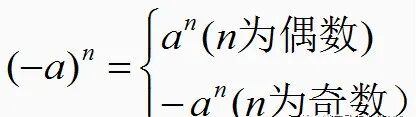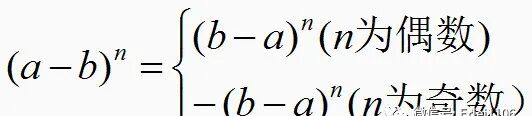# 初一数学第一单元知识点归纳

1.法则:am·an=am+n(m,n都是正整数)

(1)底数a可代单项式,也可代表多项式；

(2)运用该法则时,底数必须相同。

2.推广:am·an·ap·…·aq=am+n+p+…+q(m,n,p,…,q均为正整数)

3.逆用:am+n=am·a(m,n都是正整数)

[解析]因为a3m=8,a2n=16,所以a3m+2n=a3m·a2n=8×16=128.

4.拓展：1.法则:(am)n=amn(m,n都是正整数)底数不变,指数相乘

2.推广:[(am)n]p=amnp(m,n,p都是正整数)

3.逆用:amn=(am)n=(an)m(m,n都是正整数)

1.法则:(ab)n=anbn(n为正整数)底数分别乘方.即:积的乘方,等于把积的每一个因式分别乘方,再把所得的幂相乘

2.推广:这个性质对于三个或三个以上因式的积的乘方也适用,如(abc)n=anbncn(n是正整数)

3.利用幂的运算法则比较大小：

1.单项式与单项式相乘

(1)法则:单项式与单项式相乘,把它们的系数、同底数幂分别相乘,对于只在一个单项式里含有的字母,则连同它的指数作为积的一个因式。

(2)步骤:2.单项式与多项式相乘

(1)法则:单项式与多项式相乘,就是用单项式去乘多项式的每一项,再把所得的积相加.单项式与多项式相乘的依据是乘法分配律。

(2)字母表示:m(a+b-c)=ma+mb+m(-c)=ma+mb-mc

(3)注意事项:

①多项式与单项式相乘后仍是多项式,积的项数与多项式的项数相同.

②在计算时,不要丢掉多项式中各项前面的符号.

[分析]由于乘法运算后,所求式中各项的x的指数总是y的指数的一半,故可用幂的运算实现整体代入.

[解]-xy(x3y7-3x2y5-5y)=-x4y8+3x3y6+5xy2

=-(xy2)4+3(xy2)3+5(xy2)

3.多项式与多项式相乘

(1)法则:多项式与多项式相乘,先用一个多项式的每一项乘另一个多项式的每一项,再把所得的积相加

(2)字母表示:(a+b)(m-n)=am+a(-n)+bm+b(-n)=am-an+bm-bm

(3)注意事项:

①多项式与多项式相乘,结果仍是多项式

②在合并同类项之前,积的项数应该等于两个多项式项数之积,最后的计算结果中不能含有同类项

③把多项式与多项式相乘所得的积合并同类项后,如果结果中不含某项,那么该项的系数为0

1.同底数幂的除法

(1)法则:am÷an=am-n(a≠0,m,n都是正整数,并且m>n)

(2)推广:am÷an÷ap=am-n-p(a≠0,m,n,p都是正整数,并且m>n+p)

(3)逆用:am-n=am÷an(a≠0,m,m都是正整数,并且m>n)

2.零指数幂的意义

a0=1(a≠0),即:任何不等于0的数的0次幂都等于1.

3.单项式除法法则

4.多项式除以单项式法则

(1)法则:多项式除以单项式,先把这个多项式的每一项除以这个单项式,再把所得的商相加.

(2)字母表示:(am+bm-cm)÷m=am÷m+bm÷m+(-cm)÷m

=a+b+(-c)=a+b-c

(3)注意事项:

①多项式除以单项式,应清楚多项式的每一项的符号,相除时要带着符号与单项式相除.

②注意运算顺序,有括号的先算括号里面的.

1.平方差公式

(1)代数表达:(a+b)(a-b)=a2-b2;相同为a，相反为b.

(2)文字表述:两个数的和与这两个数的差的积,等于这两个数的平方差.

(3)重要提示:公式中的字母a和b可以是数,也可以是式子(包括单项式、多项式等).

(4)变形公式:(b+a)(-b+a)=a2-b2;(-a+b)(-a-b)=(-a)2-b2;

(-a-b)(a-b)=(-b)2-a2;

2.平方差公式的特点

(1)等号的左边是两个二项式相乘,且有两项相同,另外两项互为相反数;

(2)等号的右边是相同项的平方减去互为相反数项的平方

[解](1)(-0.1-a)(a-0.1)=(-0.1-a)(0.1+a)=(-0.1)2-a2=0.01-a2;

(2)98×102=(100-2)×(100+2)=1002-22=10000-4=9996.

1.完全平方公式

(1)代数表达:(a+b)2=a2+2ab+b2;(a-b)2=a2-2ab+b2

(2)文字表述:两个数的和(或差)的平方,等于它们的平方和,加上(或减去)它们的积的2倍

(3)变形公式:①a2+b2=(a+b)2-2ab;②a2+b2=(a-b)2+2ab;

③2ab=(a+b)2-(a2+b2);④2ab=(a2+b2)-(a-b)2;

⑤(a+b)2=(a-b)2+4ab;⑥(a-b)2=(a+b)2-4ab;

2.完全平方公式的特点

(1)等号的左边是一个二项式的完全平方的形式;

(2)等号的右边是一个二次三项式,其中有两项是平方的形式,另一项是写成平方项的两项底数乘积的2倍或—2倍.

(1)代数表达:a+b+c=a+(b+c);a-b-c=a-(b+c)

(2)文字表述:添括号时,如果括号前面是正号,括到括号里的各项都不改变符号;如果括号前面是负号,括到括号里的各项都改变符号、例 计算:(2a+b-2)(2a-b-2)

[分析]由于两个括号中含有相同的项和互为相反数的项,故可应用平方差公式计算把2a-2看作一项,b看作另一项

[解](2a+b-2)(2a-b-2)=[(2a-2)+b][(2a-2)-b]=(2a-2)2-b2

=4a2-8a+4-b2.